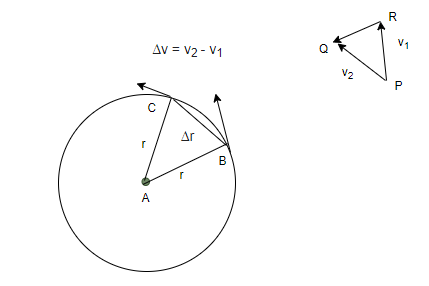# Difference Between Centripetal and Centrifugal Force

• Last Updated : 03 Dec, 2021

An object performing a circular motion is changing its velocity every second. That means a force acts on the particle which makes it move in a circular motion. While talking about this force, usually two forces are mentioned. Centripetal and Centrifugal forces are essential parts of circular motion, but both are equal and opposite in direction. An essential concept to understand while studying this motion is the difference between these two forces. Let’s look at the difference between these forces in detail.

### Centripetal Force

The figure given below shows an object which is moving in a circular motion. Assume that the speed of the object is constant. The figure shows the direction of the instantaneous velocity and points B and C. Since acceleration is the change in velocity, the change in velocity is roughly pointing towards the center of the curvature. To measure things instantaneously, the points B and C must be brought really closer and the angle between them should be infinitely small. In that, the change of velocity will point directly towards the center of the curvature.Acceleration points towards the center of the curvature, now since the velocity is continuously changing and acceleration is present. This acceleration is called centripetal acceleration ac . Here, centripetal means “center seeking” or “toward the center”. Let’s derive the equation for calculating the magnitude of the centripetal force.

The magnitude of centripetal force is given by,

F = mv2/r

This gives us the force on an object under the circular motion traveling at a speed “v” and with radius “r”. This equation depends on the square of the velocity and inversely to the radius “r”.

### Centrifugal Force

This force is also a force that acts when the object is in a circular motion. This force has the same magnitude as a centripetal force but is in the opposite direction of the centripetal force. Its unit is also Newton(N), this force does not exist when measurements are made in an inertial frame of reference, it only exists when the measurement is made with respect to the rotating frame of reference.

The magnitude of centrifugal force is given by,

F = mv2/r

This gives us the centrifugal force on an object under the circular motion traveling at a speed “v” and with radius “r”.

In real life, this force is experienced when someone is sitting inside the car taking a turn. In that situation, all the passengers experience an outwards push. This force acts there because the passengers sitting inside the car are inside the rotation frame of reference.

### Difference between Centrifugal Force and Centripetal Force

Both of these forces are essential for circular motion. While centripetal force is a real force by nature and acts in the inertial frame of reference, the centrifugal force is a pseudo force and acts in an internal frame, for example – the rotating frame of reference. Both of these forces are equal in magnitude and in opposite directions. Intuitively, the centrifugal force has a tendency to keep the object traveling in a straight line and oppose the turn, on the other hand, the centripetal force causes the object to move in a circular motion. Let’s take a look at the differences in tabular form,

### Sample Problems

Question 1: Find the centripetal acceleration on an object performing circular motion with a radius of 20m. The velocity of the object is 100m/s.

The centripetal acceleration is given by,

a = v2/r

Given:

v = 100m/s

r = 20m.

Plugging the values in the equation,

a = v2/r

⇒ a = (100)2/(20)

⇒ a = 10000/20

⇒ a = 500 m/s2

Question 2: Find the centripetal acceleration on an object performing circular motion with a radius of 2m. The velocity of the object is 10m/s.

The centripetal acceleration is given by,

a = v2/r

Given:

v = 10m/s

r = 2m.

Plugging the values in the equation,

a = v2/r

⇒ a = (10)2/(2)

⇒ a = 100/2

⇒ a = 50 m/s2

Question 3: An object(m = 8Kg) is performing circular motion with a radius of 2m. If the velocity of the object is 10 m/s, find the centripetal force acting on the object.

The centripetal acceleration is given by,

a = v2/r

Given:

v = 10m/s

r = 2m.

Plugging the values in the equation,

a = v2/r

⇒ a = (10)2/(2)

⇒ a = 100/2

⇒ a = 50 m/s2

Force acting on the object is given by,

F = ma

⇒ F = (8)(50)

⇒ F = 400 N

Question 4: An object(m = 2Kg) is performing circular motion with radius 5m. If the velocity of the object is 10 m/s, find the centripetal force acting on the object.

The centripetal acceleration is given by,

a = v2/r

Given:

v = 10m/s.

r = 5m.

Plugging the values in the equation,

a = v2/r

⇒ a = (10)2/(5)

⇒ a = 100/5

⇒ a = 20 m/s2

Force acting on the object is given by,

F = ma

⇒ F = (2)(20)

⇒ F = 40 N

Question 5: An object(m = 1Kg) is performing circular motion with radius 4m. If the centripetal force acting on the object is 100N, find the velocity of the object.

Force acting on the object is given by,

F = ma

⇒ 100 = (1)(a)

⇒ a = 100 m/s2

The acceleration of the object is given by,

a = v2/r

⇒ 100 = v2/4

⇒ 400 = v2

⇒ v = 20 m/s.

My Personal Notes arrow_drop_up